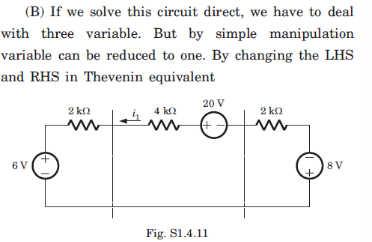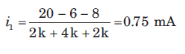Courses

# Test: Network Theorems

## 10 Questions MCQ Test Electronic Devices | Test: Network Theorems

Description
This mock test of Test: Network Theorems for Electrical Engineering (EE) helps you for every Electrical Engineering (EE) entrance exam. This contains 10 Multiple Choice Questions for Electrical Engineering (EE) Test: Network Theorems (mcq) to study with solutions a complete question bank. The solved questions answers in this Test: Network Theorems quiz give you a good mix of easy questions and tough questions. Electrical Engineering (EE) students definitely take this Test: Network Theorems exercise for a better result in the exam. You can find other Test: Network Theorems extra questions, long questions & short questions for Electrical Engineering (EE) on EduRev as well by searching above.
QUESTION: 1

###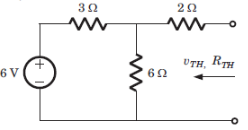Q. Vth = ?

Solution:

Vth = (6)(6)/(6 + 3) or 4 V.

QUESTION: 2

###Q. Rth = ? (in ohm)

Solution:

Rth = (3||6) + 2 or 4 ohm.

QUESTION: 3

### A battery has a short-circuit current of 30 A and an open circuit voltage of 24 V. If the battery is connected to an electric bulb of resistance 2 ohm-1, the power dissipated by the bulb is

Solution:

r = Voc/Isc = 1.2 ohm
Power used by bulb = (24 x 24) x 2/(1.2 + 2) x (1.2 + 2) or 11.5 Watt.

QUESTION: 4

Circuit for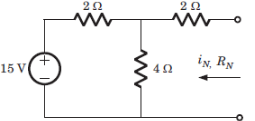Q. In = ?

Solution:

V1 = (15/2)/(1/4 + 1/2 + 1/2)
In = Isc = V1/2 = 3 A.

QUESTION: 5Rn = ? (in ohm)

Solution:

Rn = (2||4) + 2.

QUESTION: 6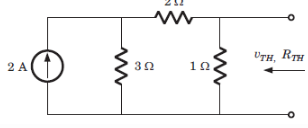Q. Vth = ?

Solution:

Vth = (2)(3)(1)/3+3 = 1V.

QUESTION: 7Q. Rth = ? (in ohm)

Solution:

Rth = 1||5 or 5/6 ohm.

QUESTION: 8

The equivalent to the given circuit is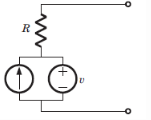Solution:

After killing all source equivalent resistance is R. Open circuit voltage is v1.

QUESTION: 9

V1 = ?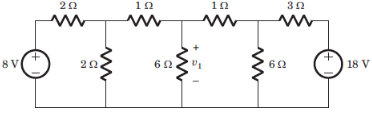Solution: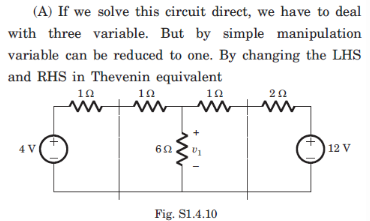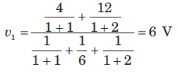QUESTION: 10

i1 = ?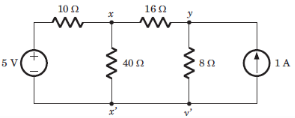Solution: